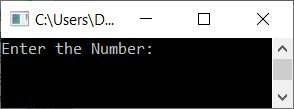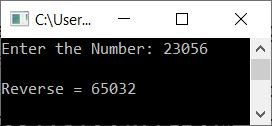# C++ Program to Reverse a Number

In this article, you will learn and get code to reverse a number entered by a user at run-time using a C++ program. Since there are many ways to create a C++ program to reverse a number, we've used some famous methods to do the task as given below:

These are the most common and popular ways to approach reversing a given number in C++. Let's start with the first way to find and print the reverse of a number.

## Reverse a number using the while loop in C++

To reverse a number in C++ programming, you have to ask the user to enter a number. Now find the reverse of that number, and then print its reverse on output as shown in the program given below:

The question is: write a program in C++ to reverse a number. Here is its answer:

```#include<iostream>
using namespace std;
int main()
{
int num, rem, rev=0;
cout<<"Enter the Number: ";
cin>>num;
while(num!=0)
{
rem = num%10;
rev = rem + (rev*10);
num = num/10;
}
cout<<"\nReverse = "<<rev;
cout<<endl;
return 0;
}```

This program was built and runs under the Code::Blocks IDE. Here is its sample run:Now supply the number, say 123, as input and press the ENTER key to reverse it and print it as shown in the snapshot given below:The dry run of the above program with user input 123 goes like this:

• Initial value, rev=0
• When a user enters the number 123, it is saved in the variable num.
• Now the condition num!=0 (of the while loop) or 123!=0 evaluates to be true, therefore program flow goes inside the loop, and all three statements gets executed.
• That is, num%10 or 123%10 or 3 gets initialized to rem.
• Then rem + (rev*10) or 3 + (0*10) or 3 gets initialized to rev. So rev=3
• And finally, num/10 or 123/10 or 12 gets initialized to num. So num=12
• Now, after executing all three statements of the while loop, its condition gets evaluated evaluated.
• That is, the condition num!=0 or 12!=0 again evaluates to be true, therefore program flow again goes inside the loop.
• Inside the loop, num%10 or 12%10 or 2 is initialized to rem once more.
• Then rem + (rev*10) or 2 + (3*10) or 2+30 or 32 gets initialized to rev. So rev=32
• num/10 or 12/10 or 1 gets initialized to num. So num=1
• Again, the condition of the while loop gets evaluated. That is, num!=0 or 1!=0 evaluates to be true, therefore all the three statements gets executed.
• That is, num%10 or 1%10 or 1 gets initialized to rem. And rem + (rev*10) or 1 + (32*10) or 1+320 or 321 gets initialized to rev. Finally, num/10 or 1/10 or 0 gets initialized to num. So after completing all three statements, we have rev = 321 and num = 0.
• Now this time, the condition of the while loop, num!=0 or 0!=0, evaluates to false. As a result, the while loop's evaluation is completed.
• We now have a variable rev that contains the reverse (321) of the given number (123).
• So just print the value of rev as the reverse of a number entered by the user at run-time.

## Reverse a Number using a for Loop in C++

This program is created using the for loop instead of the while loop. The rest of the program is identical to the previous one:

```#include<iostream>
using namespace std;
int main()
{
int num, rem, rev;
cout<<"Enter the Number: ";
cin>>num;
for(rev=0; num!=0; num=num/10)
{
rem = num%10;
rev = rem + (rev*10);
}
cout<<"\nReverse = "<<rev;
cout<<endl;
return 0;
}```

Here is its sample run with user input, 23056:## Reverse a Number Without Using the Loop in C++

This program doesn't use any type of loop, neither for nor while, to reverse a number. Let's have a look at the program first; its explanation is given later on:

```#include<iostream>
using namespace std;
int main()
{
int num, rem, rev=0;
cout<<"Enter the Number: ";
cin>>num;
CODESCRACKER:
rem = num%10;
rev = (rev*10) + rem;
num = num/10;
if(num!=0)
goto CODESCRACKER;
cout<<"\nReverse = "<<rev;
cout<<endl;
return 0;
}```

This program produces the same output as the previous program.

Note: A label named CODESCRACKER is created, on which all three statements for reversing a number are written. We used if for the condition (to check) after executing all three statements in the label CODESCRACKER. That is, if the condition evaluates to true, restart the program flow from the label using the go keyword followed by the label name.

## Reverse a Number using a User-Defined Function in C++

This program uses a user-defined function named rev() to reverse a number. It takes a number as its argument and returns its reverse.

```#include<iostream>
using namespace std;
int rev(int);
int main()
{
int num, r;
cout<<"Enter the Number: ";
cin>>num;
r = rev(num);
cout<<"\nReverse = "<<r;
cout<<endl;
return 0;
}
int rev(int n)
{
int rem, res=0;
while(n!=0)
{
rem = n%10;
res = (res*10) + rem;
n = n/10;
}
return res;
}```

## In C++, use the Class to reverse a number

This program uses classes and objects, an object-oriented feature of C++, to reverse a number given by the user:

```#include<iostream>
using namespace std;
class CodesCracker
{
public:
int rev(int);
};
int CodesCracker::rev(int n)
{
int rem, res=0;
while(n!=0)
{
rem = n%10;
res = (res*10) + rem;
n = n/10;
}
return res;
}
int main()
{
int num, r;
CodesCracker c;
cout<<"Enter the Number: ";
cin>>num;
r = c.rev(num);
cout<<"\nReverse = "<<r;
cout<<endl;
return 0;
}```

Note: An object c of type CodesCracker is created inside the main() function. Now, using object c, we've called the member function of the class CodesCracker just like normal function calls using the dot (.) operator. The function rev() also returns the reverse of a number that is passed as its argument, just like the previous program's normal function.

## Reverse a Number using an Array in C++

This is the last program on reversing a number. This program is created using an array. That is, each digit of the number gets initialized as an element of the array. And using an array, we've printed the reverse of the given number:

```#include<iostream>
using namespace std;
int main()
{
int num, rem, arr, i=0, tot=0;
cout<<"Enter the Number: ";
cin>>num;
while(num!=0)
{
rem = num%10;
arr[i] = rem;
num = num/10;
i++;
tot++;
}
cout<<"\nReverse = ";
for(i=0; i<tot; i++)
cout<<arr[i];
cout<<endl;
return 0;
}```

#### The same program in different languages

C++ Quiz

« Previous Program Next Program »